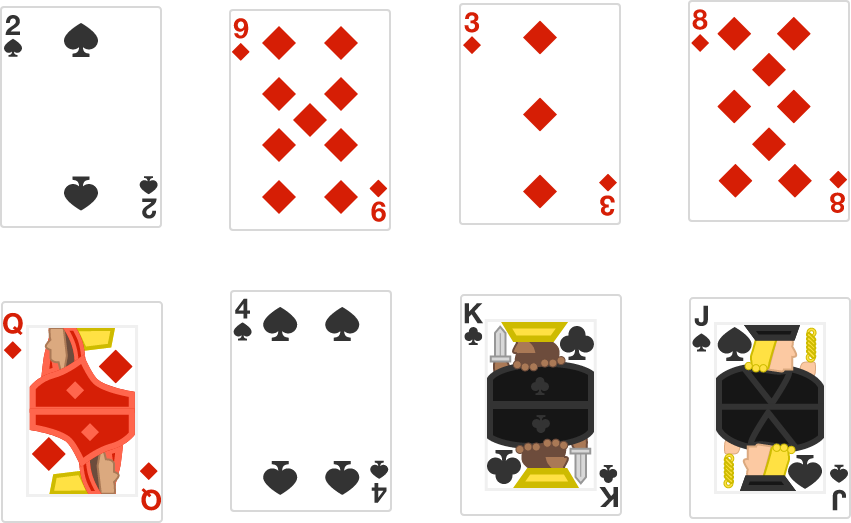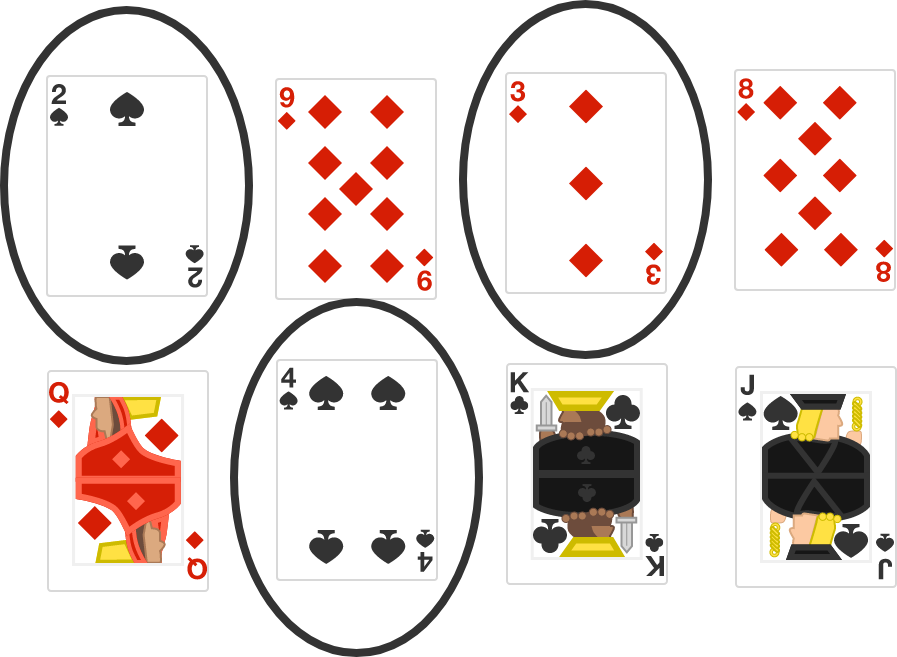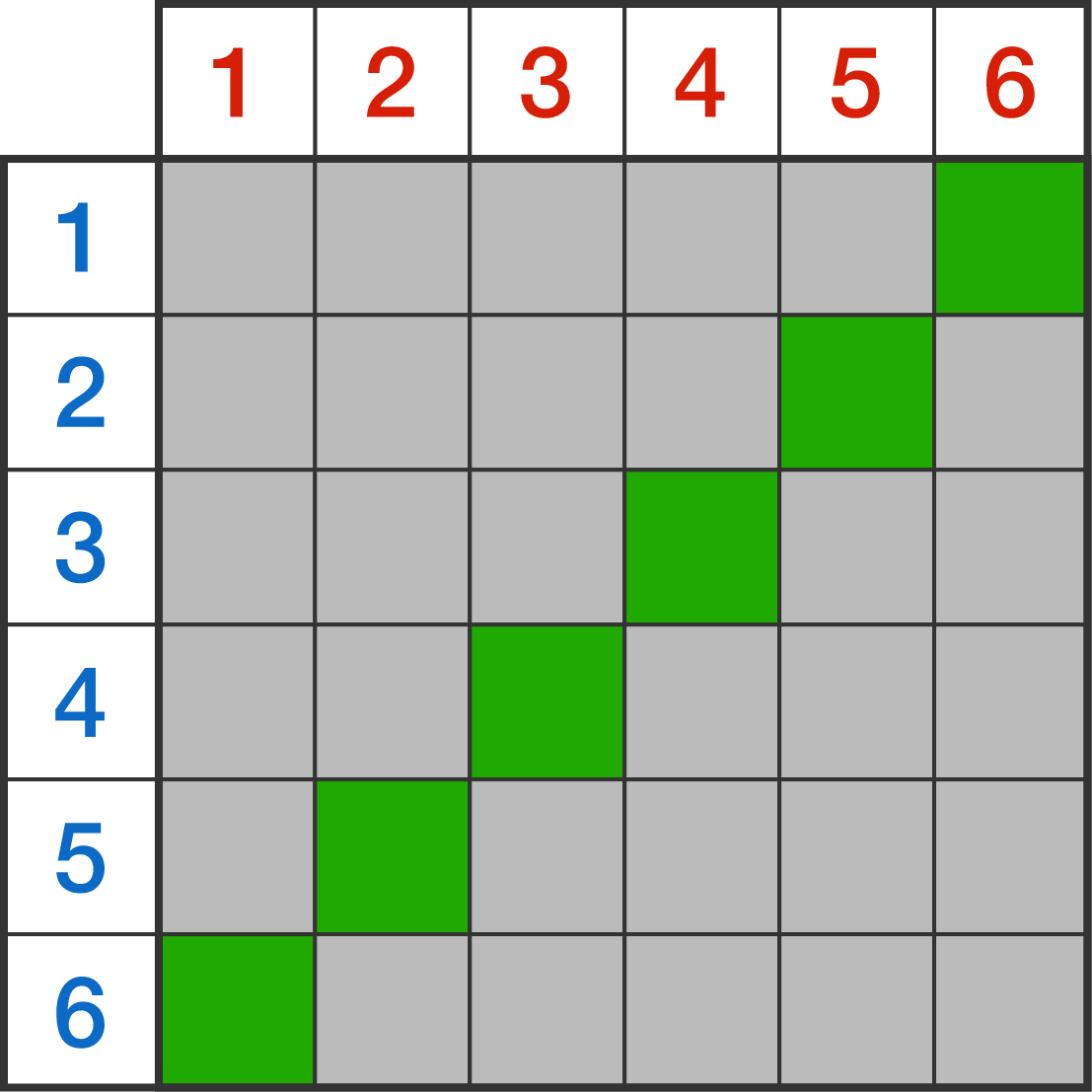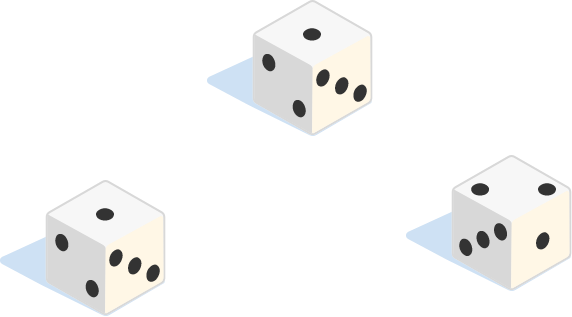### Probability FundamentalsIf you draw a card at random from the ones shown, what is the probability that the card has a number on it that is less than $\textbf6?$

# Counting and Probability

The problem you just did illustrates that probability is, in its basic essence, counting.

$\frac{ \text{outcomes that fit our condition} }{ \text{total number of outcomes overall} } = \frac{3}{8} .$You can think of the fractions from probability as expressing a pair of counting problems: how many ways can we get what we want vs. how many outcomes there are total. The fundamental theorems of probability can also be built from this basic idea, and we'll do so in this course. For now, let's try solving a few more problems by just counting; it can be more powerful than you think!

# Counting and ProbabilitySuppose you throw a pair of standard six-sided dice, one red and one blue.

Two ways to get a sum of $7$ are shown below:Including the two examples above, how many ways are there to get a sum of $7$ on the pair of dice?

# Counting and ProbabilityHow many different outcomes are possible if you're rolling the two dice from the last question and if you don't care about the sum of the outcomes?

# Counting and Probability

"What is the probability of throwing a sum of $7$ on the dice we've been using?"

we can combine the facts we just worked out.

Our desired outcome is a sum of $7:$ there are $\textbf {6}$ ways to get this sum. The total number of outcomes possible is $\textbf {36}.$

So the probability we want is $\frac{6}{36} = \frac{1}{6} .$Note it doesn't matter if we colored the dice or not! We colored them red and blue to emphasize that (for example) $3-4$ is considered different than $4-3.$ (Even when the dice look the same, they are physically different.)

# Counting and ProbabilitySuppose you throw three standard dice: what is the probability of getting a sum of $4?$ (The answers are shown with their equivalent reduced fractions when applicable.)

×